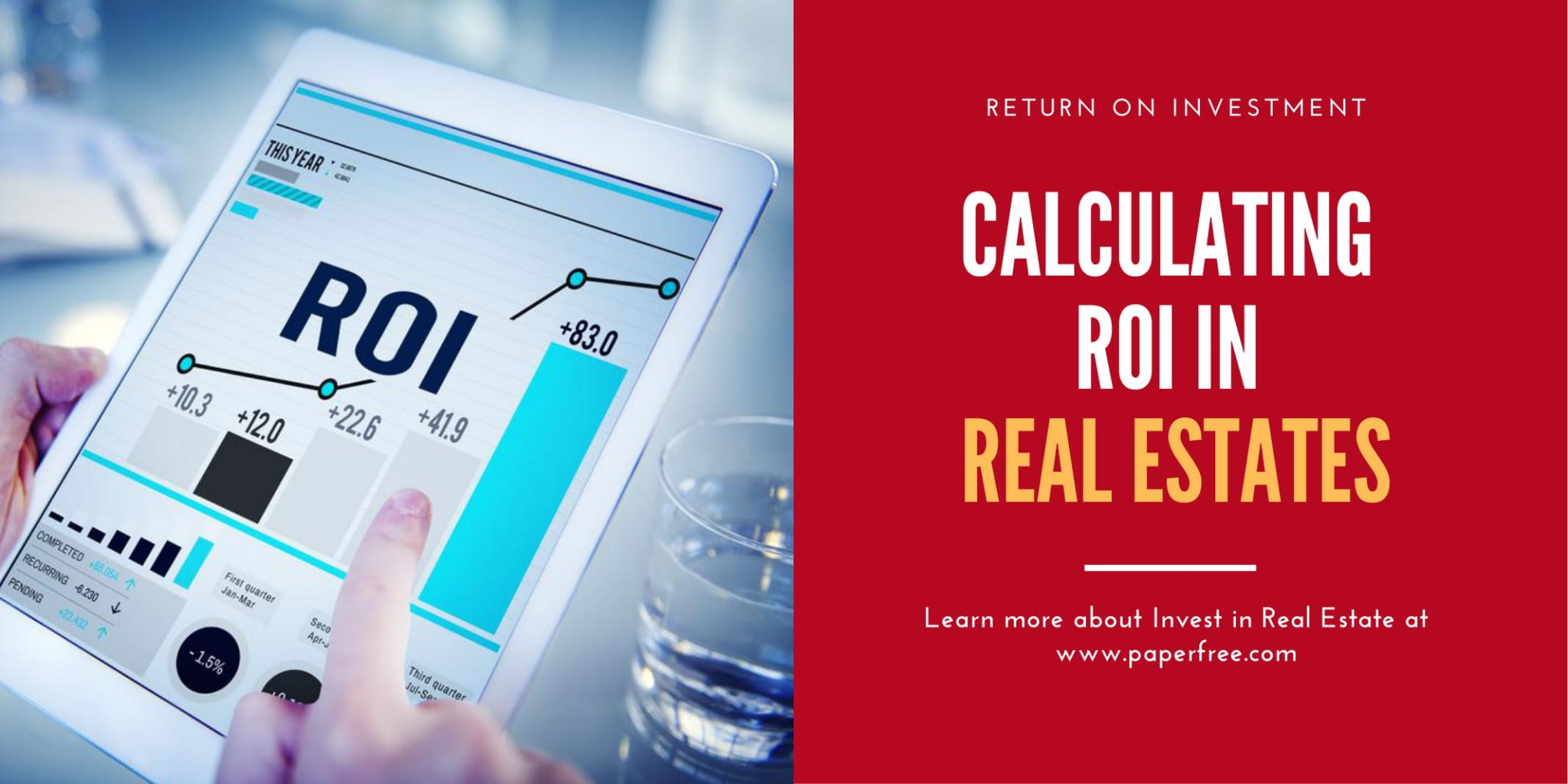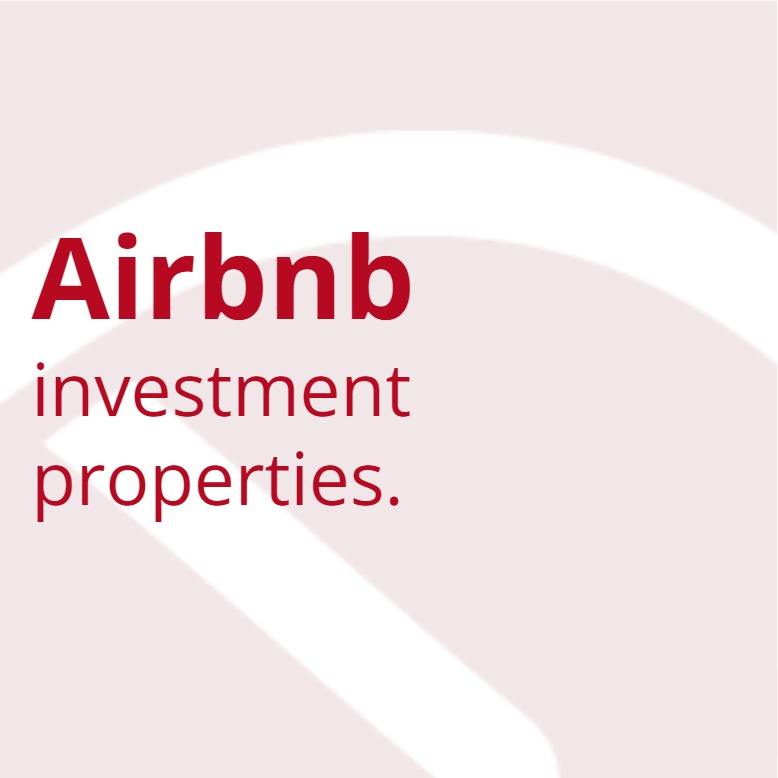### Magazine# Return on Investment (ROI) In Real Estate & how to calculate ROI

Return on Investment (ROI) In Real Estate

last update Thursday, October 01, 2020

PaperFree Tools   Investor

Real Estate Investor Tools

\$0.00 free

REQUEST NOw

In all investments such as stocks, bonds, or real estate, the goal is to make money and wealth after a certain period. If you can accurately calculate the potential profits from investing in a property before owing or acquiring it, you are more likely to succeed in doing profits. And one of the best indicators investors to use is, return on investment or ROI. If you don’t what ROI is or does, follow along.

## What is ROI in Real Estate

Return on investment is the ratio of the profits to the costs of an investment. ROI measures how much money is made on investment as a percentage of the investment’s cost. Investors use this to evaluate the profitability of an investment. Calculating ROI on a rental property is important. The return on investment represents the potential of income-generating assets. Investors who have a general idea of the rate of return can decide whether investing here is worth it or not. A higher ROI means the profits you’ll receive will be in your cost’s favor.

Not all homes or properties for sale have the same probable profits as rental properties. A beginner should use the ROI calculation when planning to buy their first rental property to compare for investment opportunities that are available in the market. By calculating ROI on investment opportunities, the new investors will be able to make smart decisions. Seasoned real estate investors also use ROI to compare different investment portfolios.

## How to calculate ROI in real estate

Now that you have an idea of ROI, you need to know the steps to calculate the ROI. Here’s how you can do it:

1. At first, estimate how much you would spend on renovation of the rental property you are investing for a year. Calculate the yearly rental income by multiplying the monthly income with 12.
2. After you’ve calculated the yearly income it’s time to subtract expenses you are expecting to incur (mortgage payments, repairing). The result of the subtraction is the cash flow.
3. Every payment that contributes to the mortgage principal will increase your property’s equity. Calculate the equity and add it to the cash flow you’ve just calculated. This generates your net income from the investment.
4. Now, follow the ROI equation and divide the net income by the total cost you paid for the investment. Follow this equation to get the ROI

ROI = (Gain in Investment - Cost of investment) / Cost of investment

Request Free
Investor Tools
Tools to underwrite deals and more
REQUEST NOW

## Limitations of ROI calculation

However, there are some limitations to the ROI calculation. While calculating the investment return is easy, some of the expenses can impact the ROI. These include maintenance, repairs, taxes, etc. Another thing that impacts the ROI calculation is the investment financing method and financing terms. Considering these complications, experts don’t recommend using the ROI formula only when you are calculating your profits. For accurate profitability, two methods can be applied.

### Calculating Cap Rate, Cost Method

The cost method in real estate helps the investor calculate a more accurate ROI for transactions done by cash. The formula for calculating ROI is by dividing the property’s net operating income by the costs related to the property purchase. Follow this equation:

Cap Rate = (Net Operating Income / Total Investment) x 100%

### 2. Calculating Cash on Cash Return, Out-of-Pocket Method

The cash on cash return is calculated by the out-of-pocket method when calculating ROI in real estate with mortgage-financed transactions. You need to list the interest you pay each month in your expenses when you buy an investment property with a loan. Dividing the annual return by the total cash invested should do the trick. Calculate the cash on cash return like this.

Cash on Cash return = (Annual Return / Total Cash Invested) x 100%

If asked about what will be a good ROI for rental property, the answers vary. Several factors influence the real estate return on investment. One most important factors are the location. When you buy a rental property you have an immediate impact on your potential profits. Another factor is the payment system. Whether you buy the property by cash or mortgage, the profits hit differently depending on the financing method.

Page keywords: Real Estate Investment , Return on Investment

What is next? Go ahead and share it!

check this out, Return on Investment (ROI) In Real Estate & Its Calculation

Want more Real Estate Investment content ? Subscribe PaperFree  Magazine!

We will send you an email with the top content on this subject: Real Estate Investment , Return on Investment.

Invest in long-life, high-quality assets around the world.